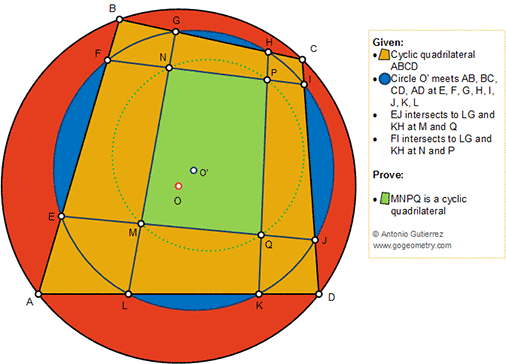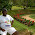## Friday, October 5, 2018

### Geometry Problem 1392: Two Cyclic Quadrilateral, Cyclic Octagon, Circle

Geometry Problem. Post your solution in the comment box below.
Level: Mathematics Education, High School, Honors Geometry, College.

Details: Click on the figure below.#### 2 comments:

1.ang: MEF + PIJ = 180, MLK + GHP = 180 => AEML, PICH cyclic
=> EML + HPI = 180

2.Let the angles of Tr. FGB be a,b,x respectively

Similarly for FGN be c,d,u
For ELA be d,e,y
For LEM be a,f,w
For JKD be g,f, 180-x
For JKQ be e,h,v
For IHP be b,g, z and
For HIC be c,h, 180 - y

Write 8 sum of angles equations for the 8 Tr.s each adding to 180.

Eliminate a,b,c,,d,e, f,g, h from these 8 equations

We end up with u + v = w + z

So each side must be = to 180 which is our desired result

Sumith Peiris
Moratuwa
Sri Lanka